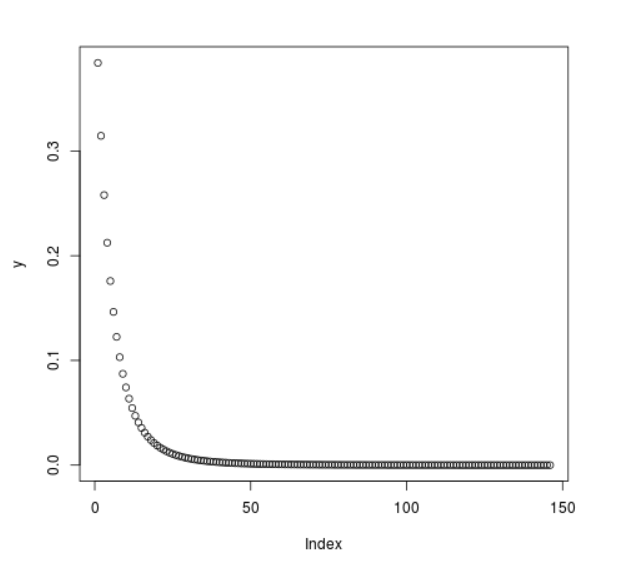Related Articles
Compute the Value of F Density in R Programming – df() Function
• Last Updated : 25 Jun, 2020

`df()` function in R Language is used to compute the density of F Distribution over a sequence of numeric values. It also plots a density graph for F Distribution.

Syntax: df(x, df1, df2)

Parameters:
x: Numeric Vector
df: Degree of Freedom

Example 1:

 `# R Program to compute ``# F Density`` ` `# Creating a sequence of x-values``x <``-` `seq(``1``, ``30``, by ``=` `2``)`` ` `# Calling df() Function``y <``-` `df(x, df1 ``=` `2``, df2 ``=` `3``)``y`

Output:

```  0.2788548009 0.0641500299 0.0255826045 0.0130822015 0.0077135607
 0.0049883063 0.0034419784 0.0024918293 0.0018719698 0.0014482499
 0.0011475506 0.0009274979 0.0007622781 0.0006355006 0.0005363874
```

Example 2:

 `# R Program to compute ``# F Density`` ` `# Creating a sequence of x-values``x <``-` `seq(``1``, ``30``, by ``=` `0.2``)`` ` `# Calling df() Function``y <``-` `df(x, df1 ``=` `3``, df2 ``=` `7``)`` ` `# Plot a graph``plot(y)`

Output:Attention reader! Don’t stop learning now. Get hold of all the important DSA concepts with the DSA Self Paced Course at a student-friendly price and become industry ready.

My Personal Notes arrow_drop_up